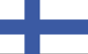﻿ Finland Distribution of family income - Gini index - Economy# Finland Distribution of family income - Gini index

Distribution of family income - Gini index: 21.5 (2015)
26.8 (2008)

Definition: This index measures the degree of inequality in the distribution of family income in a country. The index is calculated from the Lorenz curve, in which cumulative family income is plotted against the number of families arranged from the poorest to the richest. The index is the ratio of (a) the area between a country's Lorenz curve and the 45 degree helping line to (b) the entire triangular area under the 45 degree line. The more nearly equal a country's income distribution, the closer its Lorenz curve to the 45 degree line and the lower its Gini index, e.g., a Scandinavian country with an index of 25. The more unequal a country's income distribution, the farther its Lorenz curve from the 45 degree line and the higher its Gini index, e.g., a Sub-Saharan country with an index of 50. If income were distributed with perfect equality, the Lorenz curve would coincide with the 45 degree line and the index would be zero; if income were distributed with perfect inequality, the Lorenz curve would coincide with the horizontal axis and the right vertical axis and the index would be 100.

Source: CIA World Factbook - This page was last updated on January 20, 2018